# Module 8 - Forecasting

In modules five and six, we demonstrated a few steps to specify a model, calibrate the values of its parameters, and in module seven, we examined the standardized residuals – residuals diagnosis - to ensure the model proper fit with the input data.

In this module, we will take the final step and actually project a forecast: mean, standard error, and confidence interval.

In general, we are interested in forecasting the conditional mean and conditional standard deviation (aka volatility):

$$y_{T+k}=\mu_{T+k}+a_{T+k}$$ $$\mu_{T+k}=E_T [y_{T_k}]$$ $$a_{T+k}=\sigma_{T+k}\times\epsilon_{T+k}$$ $$\epsilon_{T+k} \sim \Phi(0,1)$$

Where

• $\mu_{T+k}$ is the conditional mean forecast at T+k
• $\sigma_{T+k}$ is the conditional volatility forecast at T+k

As a result; for a 95% confidence interval, the forecast is expressed as follows:

$$\mu_{T+k}-1.96\times\sigma_{T+k}\leqslant y_{T+k}\leqslant \mu_{T+k}+1.96\times\sigma_{T+k}$$

For GARCH Models, the conditional mean is constant, so the forecast procedure is primarily focused on a volatility forecast.

$$\mu-1.96\times\sigma_{T+k}\leqslant y_{T+k}\leqslant \mu+1.96\times\sigma_{T+k}$$

Using the NumXL forecast toolbar, you can generate the out-of-sample forecast values, standard errors and confidence intervals in a few steps.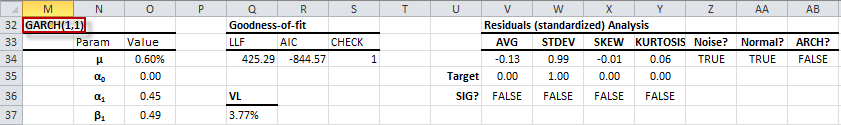1. Select the first cell in the Model (i.e. M32)
2. Locate and click on the Forecast icon in the NumXL toolbar3. The forecast wizard pops up on the screen.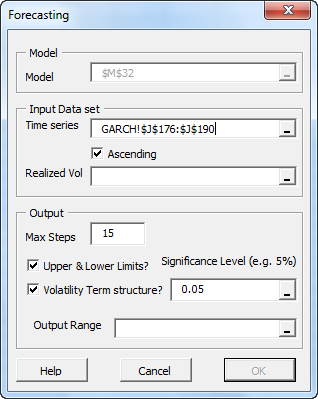4. For input data, select the cell range of the latest (i.e. most recent) weekly log returns (~ July 2012).
5. For the realized volatility, you may:
• Leave it blank, so that GARCH-fitted volatility is used, or
• Select a range of the latest weekly realized volatility (computed using a different approach)
6. In the Output -> Max Steps field, select a 15-week forecast.
7. In Output -> Volatility Term structure, leave it checked for now, as will discuss later on.
8. In the Output Range, select an empty cell in your worksheet to print the forecast formulas.
9. Click OK now.

The forecast wizard prints the formulas for different cells in the forecast table (below):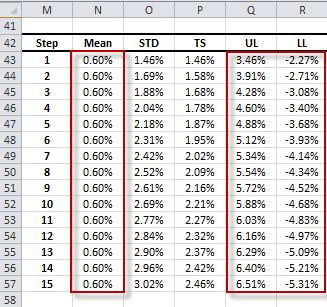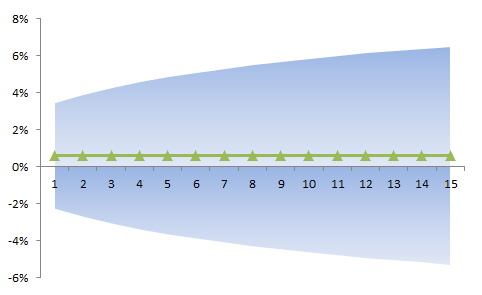Furthermore, the forecast standard error (i.e. conditional volatility) is increasing with the forecast horizon (below).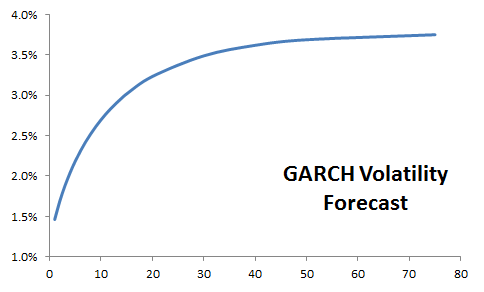In the graph above, the volatility forecast climbs to its long-run value of (3.77%).

## What are the long-run values?

For a stable time series model, the conditional mean and variance forecast converge to their long-run (historical or unconditional) values. The long-run values are implied (i.e. calculated) from the model’s parameter values.

Example: for GARCH(1,1), the long run conditional volatility (GARCH_VL) is expressed as follows:

$$\sigma_{T+\infty}=V_L= \frac{\alpha_o}{1-\sum_{i=1}^{\mathrm{max(p,q)}}(\alpha_i+\beta_i)}$$

## Term Structure

In finance, we often wish to compute a multi-period volatility forecast (aka volatility term structure).

$$\sigma_{T\to T+k}^2 = \sigma_{T\to T+1}^2 + \sigma_{T+1\to T+2}^2 + \cdots + \sigma_{T+k-1\to T+k}^2$$ $$\sigma_{T\to T+k}^2 = \sigma_{T+1}^2 + \sigma_{T+2}^2 + \cdots + \sigma_{T+k}^2$$

Now we have a base-unit of $\sigma_{T\to T+k}^2$ expressed in terms of a k-period time unit. To facilitate comparison among different periods, we use a one-period time unit for all volatility calculations:

$$\sigma_{T\to T+k}^2 = \frac{\sigma_{T+1}^2 + \sigma_{T+2}^2 + \cdots + \sigma_{T+k}^2}{k}$$

Let’s plot the GARCH volatility term structure: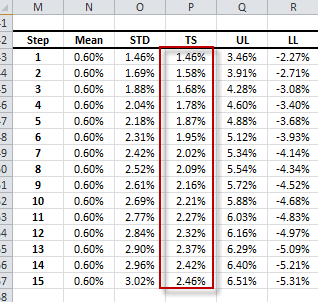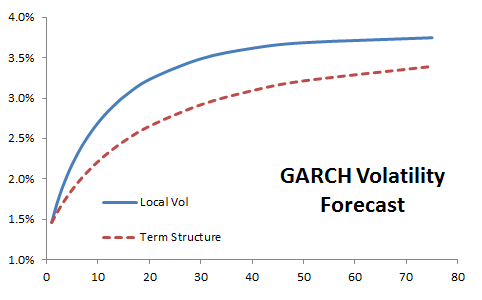As for the multi-period log returns:

$$r_{T\to T+k}=r_{T\to T+1}+ r_{T+1\to T+2}+ \cdots + r_{T+k-1\to T+k}$$

And using the same time-unit base:

$$r_{T\to T+k}=\frac{r_{T\to T+1}+ r_{T+1\to T+2}+ \cdots + r_{T+k-1\to T+k}}{k}$$

For GARCH model, the conditional mean forecast is constant ($\mu$), so the multi-period returns (term structure) are also constant ($\mu$).

## Application

Using the GARCH model earlier, what is the 3-month (12 weeks) volatility forecast per annum?

$$\sigma_{T\to T+12}^2 = \frac{\sigma_{T+1}^2 + \sigma_{T+2}^2 + \cdots + \sigma_{T+12}^2}{12}\times 52$$

This volatility value can be plugged into the Black-Scholes option pricing equation to generate a 3-month European S&P 500 index option.